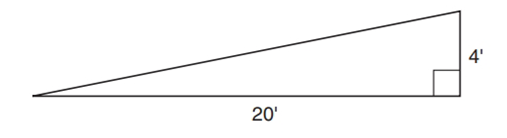Chapter 4.3, Problem 39E### Elementary Geometry for College St...

6th Edition
Daniel C. Alexander + 1 other
ISBN: 9781285195698

#### Solutions

Chapter
Section### Elementary Geometry for College St...

6th Edition
Daniel C. Alexander + 1 other
ISBN: 9781285195698
Textbook Problem
1 views

# In Exercises 38 and 39, you will need to use the square root ( ) function of your calculator.A walk-up ramp runs 20 ft horizontally while rising 4 ft. Use a calculator to approximate its length to the nearest tenth of a foot.To determine

To find:

The length of the walk-up ramp.

Explanation

Calculation:

Given:

Let the walk-up ramp denoted by ABC.

By using Pythagorean Theorem

AC2=AB2+BC2=202

### Still sussing out bartleby?

Check out a sample textbook solution.

See a sample solution

#### The Solution to Your Study Problems

Bartleby provides explanations to thousands of textbook problems written by our experts, many with advanced degrees!

Get Started

#### Change 350 ms to s.

Elementary Technical Mathematics

#### The partial derivatives ∂q∂p1 and ∂q∂p2 of the function q=5p1+4p2p1+p2.

Mathematical Applications for the Management, Life, and Social Sciences

#### Using the power series for cos x, the sum of the series is: cos(0.0625) cos(0.25) cos(0.5)

Study Guide for Stewart's Single Variable Calculus: Early Transcendentals, 8th

#### Graph each function. fx=2-lnx

College Algebra (MindTap Course List)